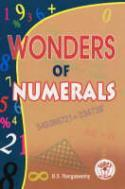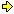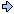World!Of
NumbersWON plate
167 |

[ November 12, 2005 ]
Wonders of Numerals
written by B.S. Rangaswamy (email)ISBN: 81-7478-492-6The first edition of this book appeared in February, 2004
by the publisher Sura Books (Pvt) Ltd., Chennai, India

[ article by yours truly PDG ]
A personal investigation in the products of two 5-digit factors
(or one 4-digit and one 5-digit) which taken together
form a pandigital (or a ninedigital) number.Last month I received a copy by the author himself. He noticed
earlier that I was interested in recreational mathematics as well
regarding topics as palindromes, powers, primes, etc. In total
57 episodes are presented. A few of them I reprogrammed with
Ubasic and I will highlight some extra interesting offshoots.
**
The first topicScintillating equations - Table (pp. 120)
The challenge here is to find all pandigital numbers
(digits 0 through 9 occurring only once) that can be expressed
as a product of two 5-digit factors so that these two factors
taken together also form a pandigital number.These are also known as pandigital Vampire Numbers and all
vampire numbers are also Friedman Numbers - thx Bruno Curfs.The computer rendered 1289 solutions... a 'prime' total !

The smallest factor from the output list happens with
1023598746 = 10482 * 97653
The largest factor from the output list happens with
6231574908 = 63102 * 98754
Note that the largest factor doesn't coincide
with the largest pandigital.

You will surely like this nice pandigital with a _unique_ solution

 1420869375 = 20481 * 69375

The largest 5-digit factor is also the right substring of the pandigital itself !

Two factors occur each four times nl. 69135 and 98640 !

 1968273450 = 28470 * 69135 2784619530 = 40278 * 69135 2819463570 = 40782 * 69135 2963817450 = 42870 * 69135

 1356497280 = 13752 * 98640 1729356480 = 17532 * 98640 2479513680 = 25137 * 98640 5142793680 = 52137 * 98640
**
Nine pandigitals can be factorized into twin sets of 5-digit
factors of which three are included inepisode 33 (pp. 20).
The remaining six twin sets are

1239756840 = 18495 * 67032
1239756840 = 23940 * 51786

1479635280 = 15864 * 93270
1479635280 = 18654 * 79320

1954283760 = 37620 * 51948
1954283760 = 37962 * 51480

2483619750 = 26475 * 93810
2483619750 = 39750 * 62481

4031985672 = 49137 * 82056
4031985672 = 58071 * 69432

4293156780 = 49260 * 87153
4293156780 = 54186 * 79230

**
I did a likewise investigation but with ninedigitals this time
expressed as a product of a 4-digit and a 5-digit factor.
The computer rendered 346 solutions !

The smallest such ninedigital is
123698574 = 2598 * 47613
The largest one can be found atepisode 26 (p.15 & 101).

The smallest 4-digit factor happens with
128943576 = 1368 * 94257
The largest 4-digit factor happens with
539184276 = 9873 * 54612
The smallest 5-digit factor happens with
135649728 = 9864 * 13752
The largest 5-digit factor happens with
317592864 = 3216 * 98754

We notice that 5-digit number 98754 is the largest factor
for the ninedigitals as well as the pandigitals. Nice coincidence !

Here also two factors occur each four times
as with the pandigitals nl. 6351 and 9864 !

 158692437 = 6351 * 24987 174582639 = 6351 * 27489 312964578 = 6351 * 49278 569837124 = 6351 * 89724

 135649728 = 9864 * 13752 172935648 = 9864 * 17532 247951368 = 9864 * 25137 514279368 = 9864 * 52137

Now the reader is invited to multiply together
these two special 4-digit factors...

6351 * 9864 = 6264_6264

An eyecatching tautonymic number ! This topic is presented

Parallel to episode 33 I compiled four ninedigital numbers
that can be factorized into twin sets of a 4- & 5-digit factor.

147963528 = 7932 * 18654
147963528 = 9327 * 15864

195428376 = 3762 * 51948
195428376 = 5148 * 37962

248361975 = 3975 * 62481
248361975 = 9381 * 26475

429315678 = 4926 * 87153
429315678 = 7923 * 54186

Note that the first one is very beautiful since the 4-digit
and 5-digit factors are 'digital anagrams' amongst themselves.

7932 * 18654
x     x
9327 * 15864
**Episode 32 : Heptads (p. 19) is a spin-off of the above searching for
10-digit numbers that have at least 7 identical digits but still
must be expressible as a 'pandigital' product of two 5-digit factors.
The following lists the smallest and largest solutions per digit.
Overall we count 113 solutions... again a 'prime' total !
Note that no solutions exist with at least seven 9's !!

digit 0 ( # 16 )
1010600000 = 10432 * 96875
6012000000 = 64128 * 93750

digit 1 ( # 11 )
1111011128 = 12403 * 89576
6111141113 = 64273 * 95081

digit 2 ( # 24 )
2262126222 = 23046 * 98157
5222222258 = 56738 * 92041

digit 3 ( # 17 )
3334733336 = 34718 * 96052
3931533333 = 54093 * 72681

digit 4 ( # 21 )
1148444444 = 13657 * 84092
5444424440 = 69524 * 78310

digit 5 ( # 10 )
1555585551 = 26403 * 58917
7555551552 = 83472 * 90516

digit 6 ( # 7 )
4666636664 = 54017 * 86392
6766616636 = 79201 * 85436

digit 7 ( # 2 )
complete set !

2777379777 = 32091 * 86547
7797777872 = 81736 * 95402

digit 8 ( # 5 )
1888388388 = 20157 * 93684
7688288888 = 80612 * 95374

digit 9 ( # 0 )
nihil

A _twofold_ solution pops up if we keep the seven identical
digits in one uninterrupted cluster.
Note that it is a very beautiful construction since the two
5-digit factors are 'digital anagrams' amongst themselves.

 5222222258 = 56738 * 920417055555558 = 76358 * 92401

56738 * 92041
x      x
76358 * 92401

Two 10-digits contain more than seven identical digits
i.e. eight identicals occurring with digit 6 in both cases
(no higher sequences exists). Quite a Beastly affair !
Concatenate the remaining digits and you'll agree with me
that 2 0 0 5 was the best year to discover this in.
My destiny with the World!Of Numbers is on schedule !

 6626666660 = 72308 * 916456666606665 = 79021 * 84365

Restricting the remaining digits to be identical leads us
finally to the next three nice solutions.

 2222323232 = 29104 * 763585553355355 = 67391 * 824056000660000 = 63750 * 94128

The third solution again reveals to us the presence of
666 or the Number of the Beast !

The extended versions of the Beast are available as well !
For instance as differences between the two 5-digit factors.

 4690873152 = 65238 * 71904and71904 – 65238 = 6666

 1802967435 = 20649 * 87315and87315 – 20649 = 66666

To conclude this expansion on the subject two equations
yielding palindromes, the first one is of a repdigit kind,
our 5-digit Beast turned topsyturvy !

 2495671308 = 47931 * 52068and47931 + 52068 = 99999

 2846031795 = 30645 * 92871and92871 – 30645 = 62226

The palindrome 62226 will come back in another format,
so keep it in mind...

**
Inspired by B.S. Rangaswamy's book I set out to look for
ninedigital numbers that are the product of two 5-digit factors
and that taken together form pandigitals.

141 solutions came up which is a palindromic total !

The smallest one is

315867942 = 15486 * 20397

The largest one is

987561234 = 28179 * 35046

The uniqueness of the following result is that
the addition of the two factors is palindromic.
And we came across that one before, didn't we...

 857264193 = 20589 * 41637and20589 + 41637 = 62226

The Beast took refuge in one of the 141 solutions !
The result is composed of the Number of the Beast and
the digitsum of 666 i.e., 6 + 6 + 6 = 18 !

 846173952 = 17082 * 49536and17082 + 49536 = 66618

**Episode 55 : Six Soldiers (p. 77)
This chapter prompts you explore 10 digit numbers having six
identical numerals positioned in a continuous line.
While recomputing all the possible solutions (total of 44),
including those with two 5-digit factors not evenly
divisible by 3, I stumbled over the following curios ¬

Particularly beautiful is this item because it
uses only two distinct digits namely '3' & '0'.
3333330000 = 34125 * 97680

Some not continuous solutions with only two distinct digits
are the following three items (from a total of 1581 - 44 or 1537).
1111551155 = 23705 * 46891
4442244242 = 45317 * 98026
5566656665 = 62473 * 89105

Also special is this item because the digits of the
tendigit number are in 'ascending order' and consecutive !
6777777888 = 72561 * 93408
**
Awesome x anagrammatical x equations
emerged while comparing various factors from
nine- and pandigital output lists

The first construct is with multiplicand 27489 and his
anagram mate 49278. The same couple of multipliers
can be applied to arrive at nine- and pandigital numbers.
Note that 27489 + 49278 = 76767 and palindromic !

 27489 * ↗↘ 5361(0) = 147368529(0)   x             x6351(0) = 174582639(0) x 49278 * ↗↘ 5361(0) = 264179358(0)   x             x6351(0) = 312964578(0)

A second construct produces a ninedigital and a pandigital with
this couple of multiplicand and multiplier anagrams.
ps. the second 49278 was also used in the above setup !
The third multiplication with the palindromic outcome
and the fourth equation with a heptadic result
finish this illustration of our four interrelated concepts
in a wonderful and astonishing manner [ Dec 4, 2005 ].

 27849 * 13506 = 376128594ninedigital   x     x 49278 * 65031 = 3204597618pandigital   x     x 94782 * 3156 = 299131992palindromic   x     x 72948 * 36015 = 2627222220heptadic

Finally three _still interesting_ leftovers from my search.
24561, 45618 & 61329 are the resp. multiplicands.
Note that in the third case the largest 4-digit multiplier
is the reversal of the smallest 4-digit multiplier.

 24561 * ↗↘ 8739(0) = 214638579(0)   x             x8793(0) = 215964873(0)

 45618 * ↗↘ 3792(0) = 172983456(0)   x             x9372(0) = 427531896(0)

 61329 * ↗↘ 7458(0) = 457391682(0)   x             x8547(0) = 524178963(0)

**
Palindromes as products of two 5-digit factors.

There are only ten palindromes consisting of nine digits
and three palindromes consisting of ten digits that are
the products of two 5-digit factors which taken together
form a pandigital. Prime factors are highlighted.

 all odd digits!393555393 385454583 690555096 431292134 707595707 629979926 919222919 966737669 all even digits!804464408 883000388 2936556392 4461771644 4878998784 = 10857 * 36249 = 13569 * 28407 = 15708 * 43962 = 16978 * 25403 = 17563 * 40289 = 19658 * 32047 = 25471 * 36089 = 25801 * 37469 = 25897 * 31064 = 28517 * 30964 = 32564 * 90178 = 51029 * 87436 = 53724 * 90816

One anagrammatical combination shows up here.

 10857 * 36249 = 393555393    x     x 15708 * 43962 = 690555096

Palindromes as products of a 4-digit and a 5-digit factor.

There are three palindromes consisting of eight digits and
thirtythree palindromes consisting of nine digits that are
the products of a 4-digit and a 5-digit factors which taken
together form a ninedigital.

 43122134 88033088 69499496 211505112 299131992 all even digits!248040842 255323552 119878911 299313992 269373962 all even digits!442000244 377434773 382676283 261999162 311676113 344202443 253000352 all even digits!460888064 286111682 226363622 543939345 262111262 424393424 236898632 207797702 232090232 253000352 625111526 528737825 261737162 520606025 524797425 806595608 586474685 all odd digits!591717195 832696238 = 1453 * 29678 = 4576 * 19238 = 5368 * 12947 = 2964 * 71358 = 3156 * 94782 see also above ! = 3418 * 72569 = 3872 * 65941 = 4197 * 28563 = 4351 * 68792 = 4378 * 61529 = 4612 * 95837 = 4629 * 81537 = 4659 * 82137 = 4926 * 53187 = 4981 * 62573 = 5473 * 62891 = 5792 * 43681 = 5824 * 79136 = 6319 * 45278 = 6518 * 34729 = 6945 * 78321 = 6982 * 37541 = 7163 * 59248 = 7528 * 31469 = 7814 * 26593 = 7864 * 29513 = 7942 * 31856 = 8231 * 75946 = 8239 * 64175 = 8297 * 31546 = 8425 * 61793 = 8475 * 61923 = 9256 * 87143 = 9341 * 62785 = 9417 * 62835 = 9526 * 87413

Five double anagrammatical combinations also show up here.

 1453 * 29678 = 43122134   x     x 4351 * 68792 = 299313992

 9256 * 87143 = 806595608  x     x 9526 * 87413 = 832696238

 4197 * 28563 = 119878911  x     x 9417 * 62835 = 591717195

 4659 * 82137 = 382676283  x     x 6945 * 78321 = 543939345

 5824 * 79136 = 460888064  x     x 8425 * 61793 = 520606025

One triple anagrammatical combination exists as well !

 2964 * 71358 = 211505112  x     x 4629 * 81537 = 377434773  x     x 4926 * 53187 = 261999162
**
Mixed anagram equations starting from a common factor.

The first and the last two constructions are remarkable in the
sense that all their factors are also anagrams among each other !

Many examples I found are displayed as well but without
the pretention of having made an 'exhaustive' list.

 4926 * ↗→↘ 53187 = 261999162palindromic   x85713 = 4222222380six_soldier   x87153 = 429315678ninedigital

 4629 * ↗→↘ 73851 = 341856279ninedigital I   x81537 = 377434773palindromic   x85173 = 394265817ninedigital II

 73851 * ↗↘ 4629(0) = 341856279(0)nine- or pandigital   x90264 = 6666086664heptadic

 3156 * ↗↘ 94278 = 297541368ninedigital   x94782 = 299131992palindromic

 28407 * ↗↘ 13569 = 385454583palindromic   x63591 = 1806429537pandigital

 62835 * ↗↘ 4197(0) = 263718495(0)nine- or pandigital   x9417 = 591717195palindromic

 90174 * ↗↘ 63825 = 5755355550heptadic   x86253 = 7777778022six_soldier

 6945 * ↗↘ 38721 = 268917345ninedigital   x78321 = 543939345palindromic

 82713 * ↗↘ 49605 = 4102978365pandigital   x54096 = 4474442448heptadic

 78321 * ↗↘ 6945 = 543939345palindromic   x40965 = 3208419765pandigital
**
Palindromic lookalikes.

Behold the next two palindromes and get enchanted.
The first equation is an 8-digit palindrome expressed
as a product of a 4- and a 5-digit factor which taken
together form a ninedigital.
The second equation is a 9-digital palindromic anagram
from the previous one except for the extra zero digit 0
expressed as a product of two 5-digit factors which
taken together form a pandigital.
A zero is also what is needed to make the crossing
from ninedigital to pandigital numbers !

8803_3088 = 4576 * 19238
8830_0_0388 = 28517 * 30964

Compare the next two palindromes and get enchanted.
The first equation is an 8-digit palindrome expressed
as a product of a 4- and a 5-digit factor which taken
together form a ninedigital.
The second equation is a 9-digit palindrome identical
to the previous one except for the extra middle digit 9
expressed as a product of two 5-digit factors which
taken together form a pandigital.

4312_2134 = 1453 * 29678
4312_9_2134 = 16978 * 25403

There exist imho no better constructions that can
synthesize this wonplate in such a beautiful way !
**
Scintillating equations with two 5-digit factors and their reversals.

Below is shown an exceptionally nice pair of equations.
The two 5-digit factors are each other's reversals.
A truly _unique_ pandigital phenomenon !

 4905361782= 52137 * 94086 ↔↕    ↕↔ 73125 * 68049= 4976083125
**

For reference goals and easy searching all the nine- & pandigitals
implicitly displayed in these topics are listed here.

Topic → 1048297653, 6231574908, 6310298754, 1420869375, 2048169375, 2847069135, 4027869135,
4078269135, 4287069135, 1375298640, 1753298640, 2513798640, 5213798640, 1849567032, 2394051786,
1586493270, 1865479320, 3762051948, 3796251480, 2647593810, 3975062481, 4913782056, 5807169432,
4926087153, 5418679230, 259847613, 136894257, 987354612, 986413752, 321698754, 635124987,
635127489, 635149278, 635189724, 986413752, 986417532, 986425137, 986452137, 793218654,
932715864, 376251948, 514837962, 397562481, 938126475, 492687153, 792354186, 1043296875,
6412893750, 1240389576, 6427395081, 2304698157, 5673892041, 3471896052, 5409372681, 1365784092,
6952478310, 2640358917, 8347290516, 5401786392, 7920185436, 3209186547, 8173695402, 2015793684,
8061295374, 5673892041, 7635892401, 7230891645, 7902184365, 2910476358, 6739182405, 6375094128,
6523871904, 7190465238, 2064987315, 8731520649, 4793152068, 4793152068, 3064592871, 9287130645,
1548620397, 2817935046, 2058941637, 2058941637, 1708249536, 1708249536, 3412597680, 2370546891,
4531798026, 6247389105, 7256193408, 274895361, 147368529, 274896351, 174582639, 492785361,
264179358, 492786351, 312964578, 2748953610, 1473685290, 2748963510, 1745826390, 4927853610,
2641793580, 4927863510, 3129645780, 2784913506, 4927865031, 947823156, 9478203156, 7294836015,
245618739, 214638579, 2456187930, 2146385790, 245618793, 215964873, 2456187930, 2159648730,
456183792, 172983456, 456189372, 427531896, 4561837920, 1729834560, 4561893720, 4275318960,
613297458, 457391682, 613298547, 524178963, 6132974580, 4573916820, 6132985470, 5241789630,
1085736249, 1356928407, 1570843962, 1697825403, 1756340289, 1965832047, 2547136089, 2580137469,
2589731064, 2851730964, 3256490178, 5102987436, 5372490816, 1085736249, 1570843962, 145329678,
457619238, 536812947, 296471358, 315694782, 341872569, 387265941, 419728563, 435168792,
437861529, 461295837, 462981537, 465982137, 492653187, 498162573, 547362891, 579243681,
582479136, 631945278, 651834729, 694578321, 698237541, 716359248, 752831469, 781426593,
786429513, 794231856, 823175946, 823964175, 829731546, 842561793, 847561923, 925687143,
934162785, 941762835, 952687413, 145329678, 435168792, 925687143, 952687413, 419728563,
941762835, 465982137, 694578321, 582479136, 842561793, 296471358, 462981537, 492653187,
492653187, 492685713, 492687153, 738514629, 462981537, 462985173, 738514629, 7385146290,
7385190264, 315694278, 315694782, 2840713569, 2840763591, 628354197, 6283541970, 628359417,
6283509417, 9017463825, 9017486253, 694538721, 694578321, 8271349605, 8271354096, 783216945,
7832106945, 7832140965, 457619238, 2851730964, 145329678, 1697825403, 5213794086, 7312568049A000167Prime Curios!Prime PuzzleWikipedia 167Le nombre 167```

```

[TOP OF PAGE]

Patrick De Geest - Belgium- Short Bio - Some Pictures